# Wythoff

## Mathematical Details

Wythoff’s kaleidoscopic construction of the uniform polyhedra is based on the idea of tiling the sphere with spherical triangles. If three mirrors are arranged so that their planes intersect at a single point, then the space between the mirrors will enclose a spherical triangle on the surface of any sphere centred on that point, and repeated reflections in the mirrors will reproduce a multitude of copies of the triangle. If the angles of the spherical triangle are chosen appropriately, the reflections will tile the sphere, one or more times. By tiling the sphere more than once, we mean that the reflections cover the whole surface a number of times before they simply repeat the positions of previous reflections. The number of times the sphere is covered is known as the density of the tiling.

A tiling of the sphere with density 1. A tiling of the sphere with density 4. Triangles have angles Triangles have angles π/5, 3π/5, π/2, π/5, π/3. π/3.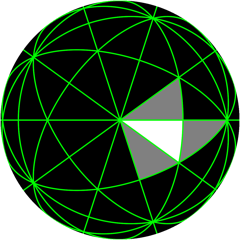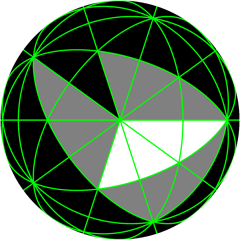The three angles of the spherical triangle will always be rational multiples of π. If the angles are π/p, π/q and π/r, the Wythoff symbol associated with the tiling contains the numbers p, q and r.

By placing a vertex point at a suitable location inside the spherical triangle enclosed by the mirrors, it’s possible to ensure that all the reflections of that point will give the vertices of a uniform polyhedron. If the spherical triangle is PQR, there are four possibilities:

1. The vertex is placed at point P. The polyhedron has the Wythoff symbol p|q r.
2. The vertex is placed at the point on PQ that bisects the angle at R. The polyhedron has the Wythoff symbol p q|r.
3. The vertex is placed at the incentre of PQR, the point that lies on the bisectors of all three angles. The polyhedron has the Wythoff symbol p q r|.
4. The vertex is placed at the point that, when rotated around any of the triangle’s corners by twice the angle at that corner, is displaced by the same distance in each case. (This distance is the length of an edge of the polyhedron.) Only even-numbered reflections of the original vertex are used. The polyhedron has the Wythoff symbol |p q r.
(1a) Vertex positions for polyhedron with Wythoff symbol 2|3 5 (1b) Polyhedron with Wythoff symbol 2|3 5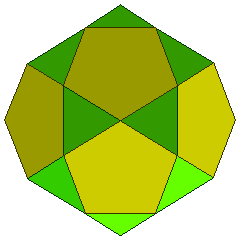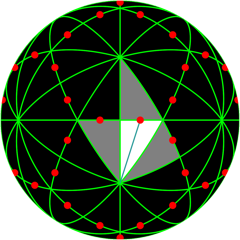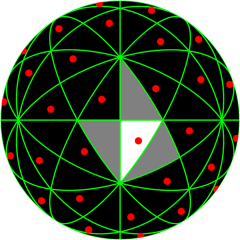By applying these four choices to all the possible triangular tilings of the sphere, 79 of the 80 uniform polyhedra can be constructed; the exceptional, non-Wythoffian case is described in Har’El. The count of 80 includes a single representative from each of the five infinite classes of prisms and antiprisms. A prism can be constructed with regular polygons of any number of sides for two of its faces, and squares for all the other faces; variations on this basic idea give the other four classes.

Reference: Zvi Har’El, “Uniform Solution for Uniform Polyhedra”, Geometriae Dedicata 47: 57–110, 1993.Applets Gallery / Wythoff (Technical Notes) / created Saturday, 19 January 2002 / revised Sunday, 20 January 2002
If you link to this page, please use this URL: https://www.gregegan.net/APPLETS/26/WythoffNotes.html
Copyright © Greg Egan, 2002. All rights reserved.